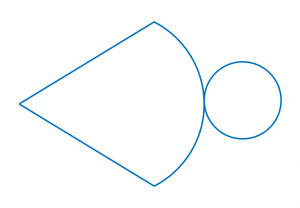# 8.2.2 Solid Geometry (III), PT3 Focus Practice

Question 6:
Puan Rafidah wants to prepare a right circular cone shaped container in order to fill it with candies for her family Hari Raya Celebration. Diagram below shows the net of a right circular cone.The circumference of the lid is 44 cm and its height is 24 cm.
Calculate the volume, in cm3 , of the container.
$\left[\pi =\frac{22}{7}\right]$

Solution:

Question 7:
A barrel contains 36 l of mineral water. ⅔ of the mineral water is mixed with 6 bottles of syrup, with a volume of 1.5 l each. The drink will be poured into a number of bottles with the same volume of 300 ml.
How many number of bottles needed?

Solution:

Question 8:
A spherical balloon expands such that its volume increases from 36π cm3 to 288π cm3 .
Find the increment of the radius, in cm, of the balloon.

Solution:

Question 9:
A boy is given three pieces of cards by his teacher. The cards consist of one rectangle card and two circle cards of the same size as shown in the diagram below. The circumference of each circle is 66 cm.He is required to combine the cards to form a right circular cylinder.
Calculate the volume, in cm3, of the right circular cylinder.

Solution:

Question 10:
Diagram below shows cube P and cube Q with the total surface area of 726 cm2 and 1014 cm2 respectively.Find the difference in volume between cube P and cube Q.

Solution:

# 8.2.1 Solid Geometry III, PT3 Practice

 
 
8.2.1 Solid Geometry III, PT3 Practice
 

  Question 1:
 
The diagram below shows a cone with diameter 14 cm and height 6 cm.
 

   
Find the volume of the cone, in cm3.
 

 
Solution:
    $\begin{array}{l}\text{Volume of a cone}\\ =\frac{1}{3}\pi {r}^{2}h\\ =\frac{1}{\overline{)3}}×\frac{22}{\overline{)7}}×{7}^{\overline{)2}}×\overline{)6}2\\ =308{\text{cm}}^{3}\end{array}$

 

 
 
 
 
Question 2:
 In the pyramid shown, the base is a rectangle.
 

   

If the volume is 20 cm2, find the height of the pyramid, in cm.
 
 

  Solution:
 
  $\begin{array}{l}\text{Volume of a pyramid}=\frac{1}{3}×\text{base area}×h\\ \frac{1}{3}×\text{base area}×h=20\\ \frac{1}{3}×5×4×h=20\\ \text{}\frac{20}{3}×h=20\\ \text{}h=\overline{)20}×\frac{3}{\overline{)20}}\\ \text{}h=3\text{cm}\end{array}$

 
 
 
 
 
Question 3:
 
Diagram below shows a composite solid consisting of a right circular cone, a right circular cylinder and a hemisphere.
 

 
   

The volume of the cylinder is 1650 cm3. Calculate the height, in cm, of the cone.
 
   
 
 

  Solution:
 
  $\begin{array}{l}\text{Volume of a cylinder}=\pi {r}^{2}h\\ \frac{22}{7}×{r}^{2}×21=1650\\ \text{}{r}^{2}=\frac{1650×7}{22×21}\\ \text{}=25\\ \text{}r=5cm\\ \text{Thus the height of the cone}\\ =39-5-21\\ =13cm\end{array}$

 
 
 
 
 
Question 4:
 
The cross section of the prism shown is an isosceles triangle.
 

   
The volume of the prism, in cm3, is
 
 

  Solution:
    $\begin{array}{l}\text{Height of the}△=\sqrt{{10}^{2}-{6}^{2}}\\ \text{}=\sqrt{64}\\ \text{}=8cm\\ \\ \text{Volume of prism}\\ =\text{Area of cross section}×\text{Length}\\ =\left(\frac{1}{\overline{)2}}×{\overline{)12}}^{6}×8\right)×16\\ =768c{m}^{3}\end{array}$

 

 
 
 
 
Question 5:
 
A right circular cone has a volume of 77 cm3 and a circular base of radius 3.5 cm. Calculate its height.
 
 

  Solution: